«Актуальные вопросы в сфере социально-экономических, технических и естественных наук и информационных технологий» (3-4 апреля 2014г.)

Viter M. E., Berdnik M. G., Bondar O. E.

Oles Honchar Dnipropetrovsk National University

PREDICTING FOREIGN CURRENCY SAVINGS OF UKRAINIAN POPULATION USING PARTIAL DIFFERENTIAL EQUATIONS

Forecasting cash savings of a family plays a major role in any state. On the basis of these data a customer basket is formed, the purchasing power of the population is determined, the demand for certain groups of goods a family can afford is calculated depending on their level of income and expenses, the minimum wages are calculated to control the required minimum level of welfare of families, etc.

Using the known stochastic parabolic equations of cash savings  for the function of accumulated savings in foreign currency of Ukraine’s population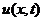, and the value of distribution density function of households’ accumulated savings in foreign currency of Ukraine’s population at the initial time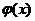, the Cauchy problem for partial differential equations for foreign currency savings of Ukraine’s population from the time was formulated in this way: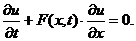(1)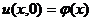,(2)

where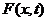is cash savings rate,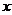is the total amount of money,is time,is the function of density distribution of families’ savings in foreign currency of Ukraine’s population at the initial moment.

According to data from the website of the State Statistics Committee , the regression model of the dependence of savings in foreign currency of Ukraine’s population on timewas constructed.is determined with a function (functional dependence of the cash savings in foreign currency of the population of Ukraine on time and money). Ifis responsible for the rate of accumulation, then.

The two cases were considered: linear regression model and a quadratic regression model. Both models are adequate to the observed data by Fisher criterion.

Using data over the 2008–2010 years, the nonlinear regression of cash savings, depending on time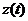was built. We got a quadratic regression model of the following form: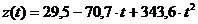(3)

To construct the distribution density function of families’ savings in foreign currency of Ukraine’s population at the initial time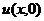the statistics  was used. Functionis based on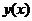(functional dependence of the number of families on the money).

Using the known stochastic parabolic equations of cash savings (1) under certain trend for accumulation function of families and families’ accumulation distribution function at the initial time (2), the Cauchy problem was formulated for the first order of partial differential equations.

Using the well-known method of the solution of the Cauchy problem of partial differential equations, the analytical solution of this one was obtained.

This analytical solution allowed us to find the density distribution of households according to time and the amount of savings. To automate the calculation offunction the program was developed in the programming language C# within the Microsoft Visual Studio 2010 environment. With the developed program the density distribution of Ukrainian households after 2008 was calculated.

The list of references:

1. Erofeenko V. T. Partial differential equations and mathematical models of the economy / V. T. Erofeenko, I. S. Kozlovskaya. – M.: Editorial URSS, 2004. – 248 p.

2. Electronic resource. – Mode of access: http://www.ukrstat.gov.ua